# Ghost Carpet Fractal

### Ghost Fractal:

This is a fractal because through each new level, the same thing is continuously done. In the case of my fractal, after each level each squares size is divided by 4, while also removing the the same points that would correspond in the new square. The points that I removed from my fractal are (0,0), (4,0), (0,4) and (4,4), and (1,1).

### Final Prints: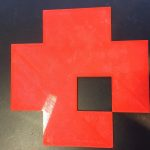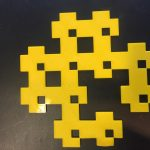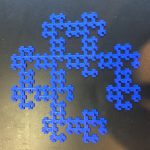### First Calculation of Area:

To calculate area for this fractal you would do the following…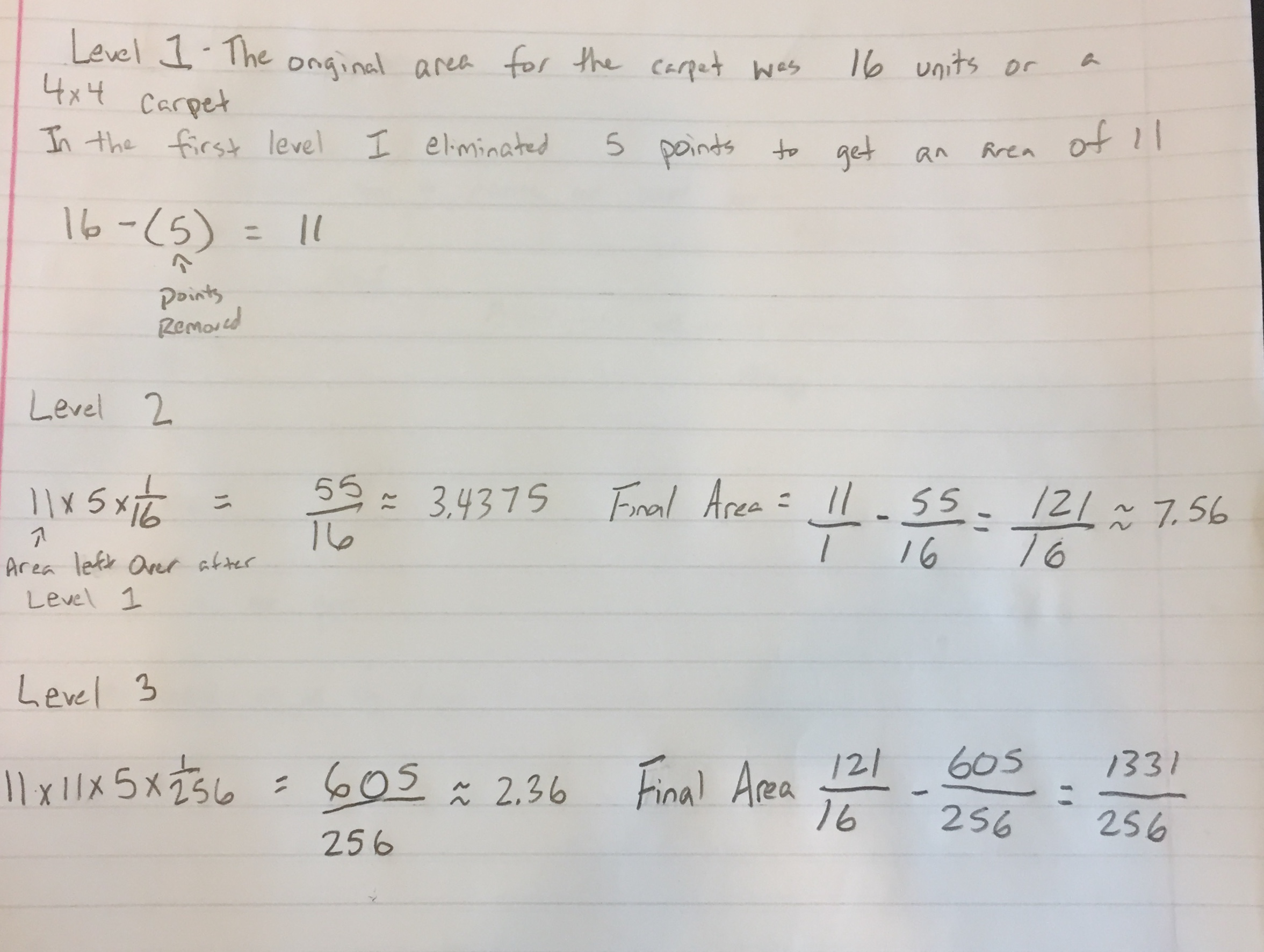In the level 2, the 11 is how many squares will be kept, and the 5 is how many are subtracted away for each cube. In the level 1 carpet each square represented 1 full units. Now each square represents 1/16th of a unit which is why you multiply by that fraction to get the 55/16 which is the amount that will be subtracted away from the original 11 units of the level 1 fractal.

However in the level 3, you would do the same math for the level 2 but then just multiply by an additional 11 for the new level. The total amount each unit would be worth now would be 1/256. So the final area left over after the level 3 carpet would be 1331/256 which is equivalent to 5.199

### Geometric Series Calculations: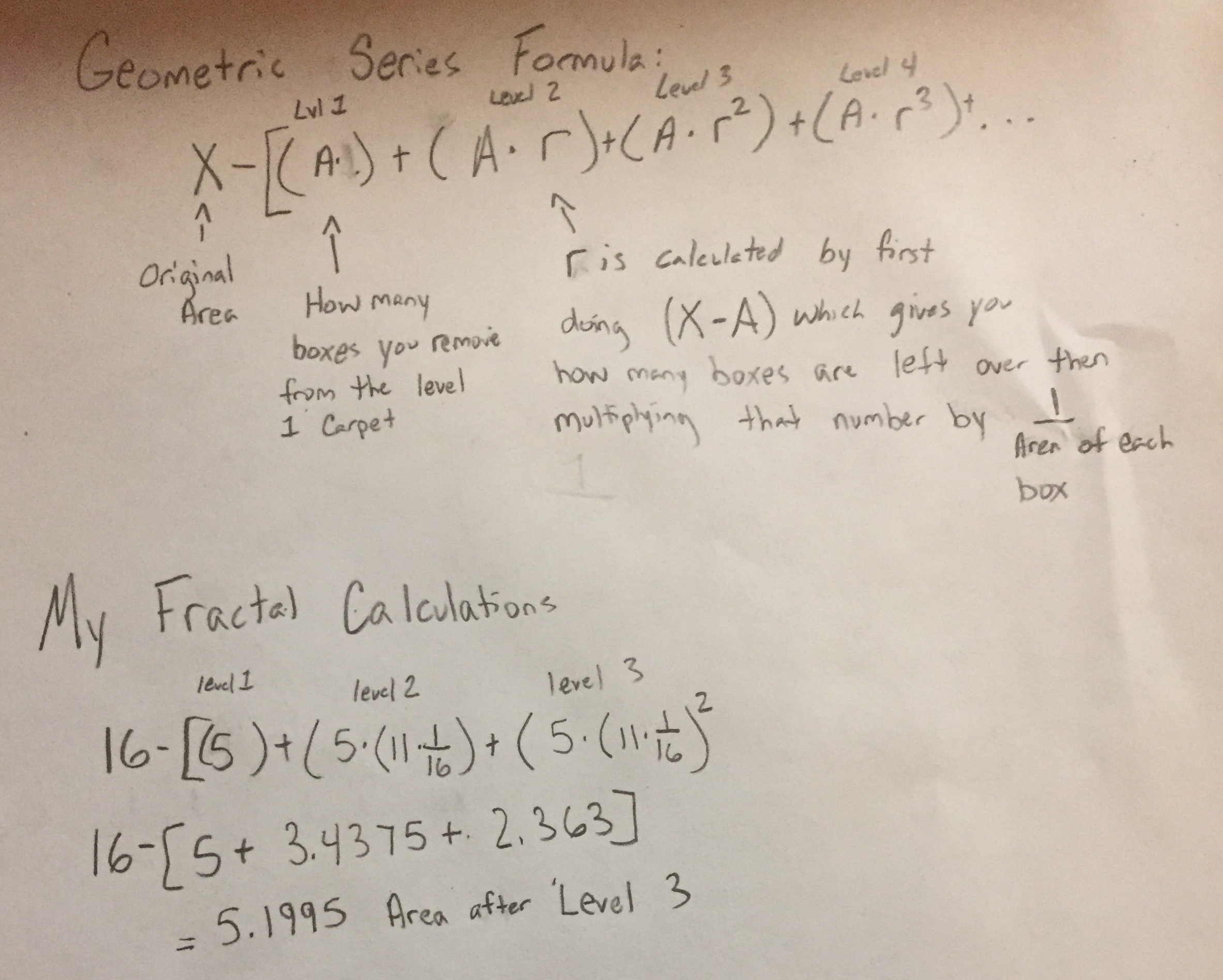The last calculation is to determine whether or not the fractal will have a finite or infinite area after continuously adding new levels. This is done by using the formula  A* (1/(1-r)). Where just like in the above A=5 and R=0.6875 calculated by doing 11*1/16. If R<1 then the area should be finite, however when we plug the numbers into the formula 16(original area)-5*(1/(1-.6875)) we actually get a final answer of 0. This is because although the final area is 0, there are still points within the fractal that will ultimately never get erased entirely. So within the square, there would be a bunch of random points without anything actually connecting them together.

### Dimension Calculations:

When calculating the dimensions, you first have to find out how much you scale down the original fractal to get to the new piece in the level 2. In the level one, a whole side was equal to 1 but in the following level that was then cut into 4 separate pieces so the scaling fraction would be 1/4. Next you must figure out how many copies you must scale down, which in the case of my fractal since I eliminated 5 pieces from the 16 I would get a total of 11 copies which would be 1/11. Now to put this into the dimension formula which is.  A^D=B and  when you plug in the numbers you would get…

(1/4)^d=1/11           4^d=11=D and ultimately the dimension would equal 1.7297